# Discover How to Play Bunco with Excel – Use Excel RAND Function to Design Dice Games

## Bunco on Excel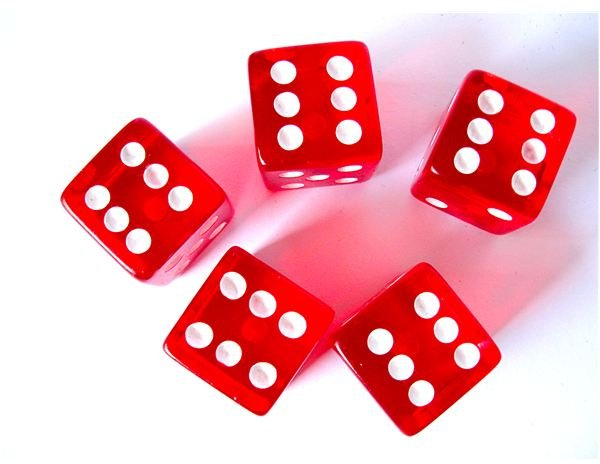Problem: Your kids are bored waiting somewhere. Pull out your laptop and build a quick Bunco game to keep them occupied.

Even if you’re not a Bunco fan, you can use the following method to create other types of dice games in Excel. (For another example, see Play Craps with Excel.)

Image Credit: morgueFile/alvimann

## Creating a Bunco Game

Strategy: Without using macros, you will have to use the manual calculation mode and a single iteration mode to allow the worksheet to keep track of scoring. Follow these steps.

1) From the menu, select Tools – Options – Calculation.

2) In the Calculation section, choose Manual. Uncheck the option to Recalculate Before Save. In the Iteration section, choose the Iteration checkbox. Change both parameters to 1, as shown in Fig. 307. (Click any image for a larger view.)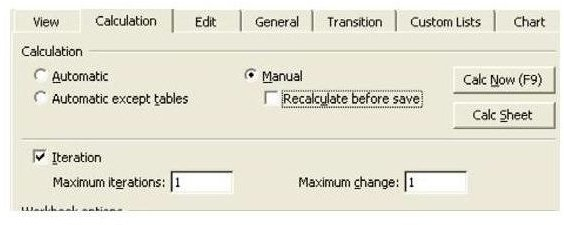Manual calculation with iteration will allow spreadsheets with circular references to actually work. Every time that you hit the F9 key to calculate the worksheet, the cell with a circular reference will recalculate on the basis of the previous value of the cell.

3) Follow the steps from the article Play Dice Games with Excel to build three dice in B2, D2, and E2, as shown in Fig. 308.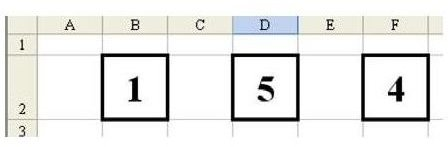4) In cell C5 enter the label “Round”. In cell C6, enter the label “This Score”. In cell C7, enter the label “Total Score”. Right-justify each label using the button on the Standard toolbar, as shown in Fig. 309.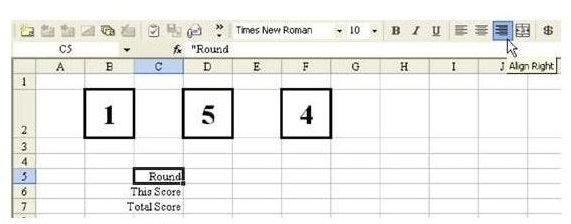Bunco is played in six rounds. During your turn, you roll three dice. For each dice that matches the Round #, you get 1 point. If you happen to roll three matching dice that do not match the round number, you get 5 points. If you roll three dice that match the round number, you get 21 points. On any turn where you get no points, you then advance to the next round.

5) For now, enter the number 1 in D5.

The formula for the score in D6 is fairly complex. You can break down the rules as follows:

• If B2, D2, and F2 all match, and they match the round number in D5, then you get 21 points.

• Otherwise, if B2, D2, and F2 all match then you get 5 points.

• Otherwise, add one point if B2 matches D5. Add one point if D2 matches D5. Add one point if F2 matches D5.

You will have to nest a couple of IF functions. The syntax of the IF function is: =IF(Some Test, Value if True, Value if False). If the test portions of the IF statements have several conditions that must be met, then you should include all of the conditions as arguments of the AND function.

Our first scoring rule is “If B2, D2, F2, and D5 all match, then 21 points”. The AND statement for this is =AND(B2=D2,D2=F2,F2=D5). The IF statement to give 21 points would be =IF(AND(B2=D2,D2= F2,F2=D5),21,___).

The next scoring rule replaces the ___ in the third parameter of the IF function. This scoring rule says that if B2, D2, and F2 match, then you get 5 points. The AND statement would be AND(B2=D2,D2=F2). This portion of the IF statement would be =IF(AND(B2=D2,D2=F2), 5,___).

The final scoring rule says that for any of the dice that match the round number, you get 1 point. This actually requires three new IF functions, added together: IF(B2=D5,1,0)+IF(D2=D5,1,0)+IF(F 2=D5,1,0).

## Creating a Bunco Game (Continued)

6) Nesting all of these together, you get the following formula. Enter it in cell D6.

=IF(AND(B2=D2,D2=F2,B2=D5),21,IF(AND(B2=D2,D2=F2),5,IF(B2=D5,1,0)+IFD2=D5,1,0)+IF(F2=D5,1,0)))

7) Hit the F9 key until you get three dice that match, as shown in Fig.310. Check that the scoring for This Score works as planned.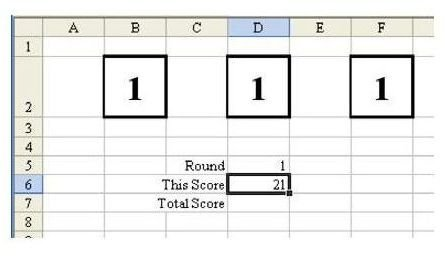8) Hit F9 again until you have a setting where This Score is 0. This is the tricky part. Before you can enter the circular reference formula, you need to enter the initial value for the cells in the worksheet.

9) In cell D5, enter a –1.

10) In cell D7, enter the number 0, as shown in Fig. 311.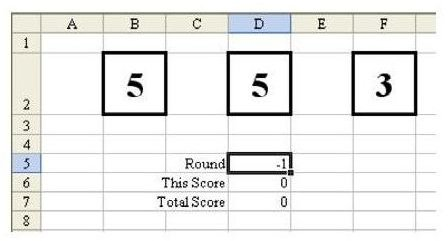11) In cell D7, enter the formula =D6+D7. This formula will add the score from This Score to the Total Score.

12) In cell D5, enter the formula =IF(D6=0,1+D5,D5). This gives you a formula that will increment the round number any time that the dice produce zero points. When you enter this formula, because D6 is initially 0, the –1 will change to a 0.

13) Immediately Save and Close the game after entering these formulae. It is important that Excel NOT CALCULATE before saving. That is why you unchecked the Calculate Before Save option in Fig. 307.

Result: To play a round of Bunco, hit the F9 key to roll the three dice, as shown in Fig. 312. When the game is complete, close the worksheet WITHOUT SAVING. Open the worksheet to start a new game.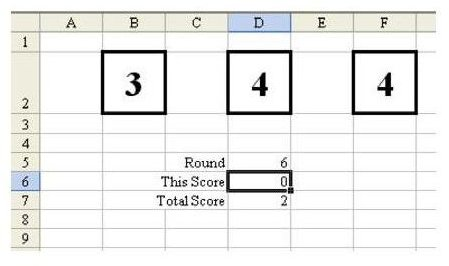Gotcha: Before entering the numbers in D5, make sure that the dice do not all match.

Summary: Using manual calculation mode, you can create simple dice games.

Commands Discussed: Manual Calculation

Functions Discussed: =RAND(); =IF(); =AND()

## More Excel Resources

If you’re looking for other tips and tutorials, be sure to check out the hundreds of other Microsoft Excel articles available at Bright Hub. In particular, you may be interested in the following collections.

91 Tips for Calculating with Microsoft Excel – Taken from the book Learn Excel from Mr. Excel, this collection of tips focus on the wide array of calculation tools found in Microsoft’s popular spreadsheet application. Become an Excel expert in no time!

Excel Formatting Tips from Mr. Excel – This set of 72 tips offers strategies and advice on how to use Excel’s formatting capabilities to better organize and analyze data.